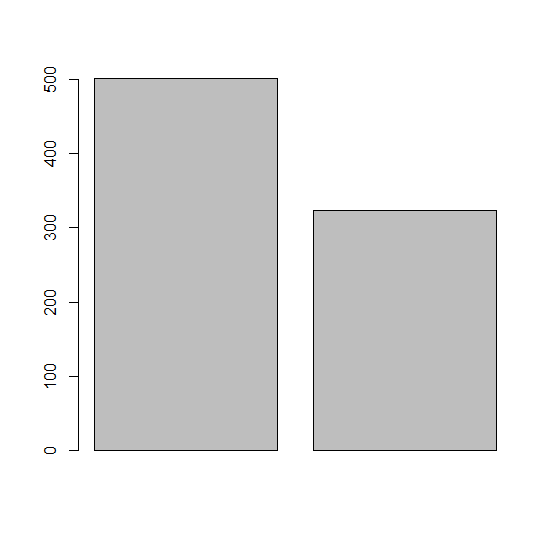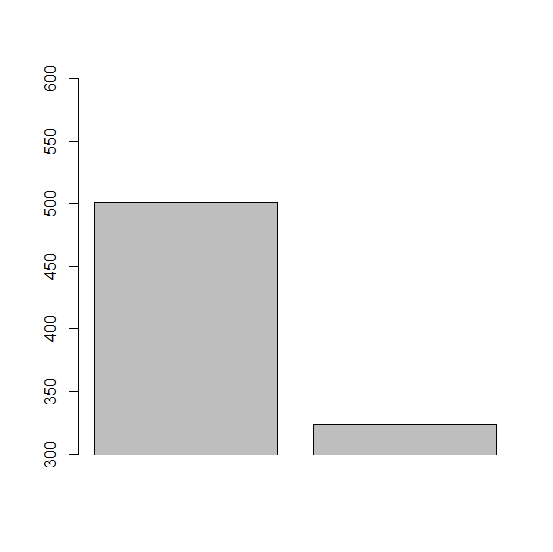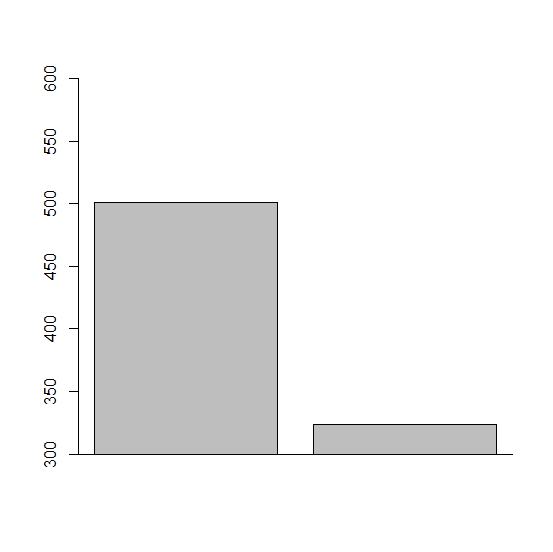# How to create bar plot in base R with different limits for Y-axis?

To create a bar plot in base R with different limits for Y-axis, we can use ylim argument but generally that behaves badly, such as extending the bars below X-axis. Therefore, we need to fix those things. Check out the below example to understand how it can be done.

## Example

> x<-c(501,324)
> barplot(x)

## Output## Example

> barplot(x,ylim=c(300,600))

## Output## Example

> barplot(x,ylim=c(300,600),xpd=FALSE)

## Output## Example

> box(bty="l")


## Output